# Spikes¶

Title
Spikes Element
Dependencies
Matplotlib
Backends
Matplotlib
Bokeh
In :
import numpy as np
import holoviews as hv
from holoviews import opts

hv.extension('matplotlib')Spikes represent any number of horizontal or vertical line segments with fixed or variable heights. There are a number of different uses for this type. First of all, they may be used as a rugplot to give an overview of a one-dimensional distribution. They may also be useful in more domain-specific cases, such as visualizing spike trains for neurophysiology or spectrograms in physics and chemistry applications.

In the simplest case, a Spikes object represents coordinates in a 1D distribution:

In :
xs = np.random.rand(50)
hv.Spikes(xs).opts(alpha=0.4, spike_length=0.1)

Out: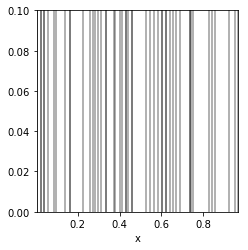We can overlay Spikes on top of other Chart elements (such as Points or Curve) if we want to draw attention to where samples are along the x-axis:

In :
ys = np.random.rand(50)
points = hv.Points((xs, ys))
spikes = hv.Spikes(xs)
points.opts(color='red') * spikes

Out: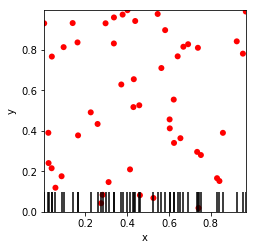When supplying a second dimension to the Spikes element as a value dimension, these additional values will be mapped onto the line height. Optionally, it is also possible to map dimensions to style options. This way we can, for example, plot a mass spectrogram:

In :
hv.Spikes(np.random.rand(20, 2), 'Mass', 'Intensity').opts(cmap='Reds', color='Intensity')

Out: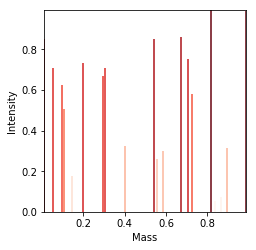Another possibility is to draw a set of Spikes offset by a position, which can be useful for plotting discrete events like neurons firing. Here we generate 10 separate random spike trains and distribute them evenly across the space by setting their position. By declaring some yticks, each spike train can be labeled individually:

In :
overlay = hv.NdOverlay({i: hv.Spikes(np.random.randint(0, 100, 10), 'Time').opts(
position=0.1*i, spike_length=0.1) for i in range(10)})

ticks = [((i+1)*0.1-0.05, i) for i in range(10)]

overlay.opts(show_legend=False, yticks=ticks)

Out: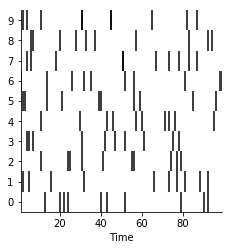Finally, we may use Spikes to visualize marginal distributions as adjoined plots using the << adjoin operator:

In :
points = hv.Points(np.random.randn(500, 2))
(points << hv.Spikes(points['y']) << hv.Spikes(points['x'])).opts(
opts.Spikes(alpha=0.2))

Out: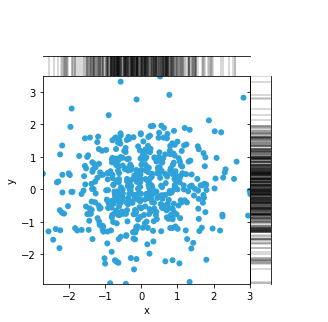For full documentation and the available style and plot options, use hv.help(hv.Spikes).

Download this notebook from GitHub (right-click to download).